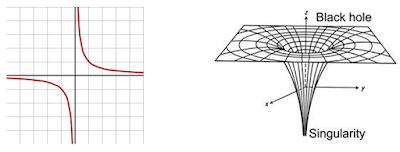### Section 1.7 - The Theory of Everything

#### Unifying Physics

It seems like between relativity and quantum mechanics, we've basically got everything figured out. Both models have been experimentally proven and are predictive to unbelievable accuracy. The problem is, we haven't been able to reconcile the two (check out this article

Here's the problem: the two are mutually incompatible for a number of mathematical reasons. Essentially, relativity relies on a notion of continuous spacetime, while quantum mechanics relies on discrete states.

Normally when we're calculating things on the quantum scale, we ignore gravity because it's so weak compared to the other forces. But many physicists are working to understand the singularity of a black hole or the point of the Big Bang, when the Universe began (more on this in the astrophysics unit). These points have enormous gravity that cannot be ignored, so we need to merge the two

These are points of infinite density (a given mass over an infinitely small point-volume). Equations cannot handle infinities in their calculations, so the models fail to be predictive.A mathematical singularity and the singularity of a black hole

There are a number of theories proposed that may be able to unify the two theories. For starters, we haven't been able to come up with a quantum theory of gravity. This quantum gravity would require the existence of some yet undiscovered particle (a "graviton") that mediates gravity the same way the other bosons mediate the other forces

Other physicists propose what's known as "string theories" in higher dimensions that can unify the forces. The idea is that the concept of point-like particles can be thought of as vibrations of one-dimensional strings throughout the Universe. Some think it's the future of physics, others think its hogwash. If you're ambitious, trying read Hyperspace by Michio Kaku and decide for yourself!

Regardless, the unification of physics would be the greatest theoretical breakthrough in history. Einstein and Hawking both tried unsuccessfully, but perhaps new genius awaits